# find the equation of the tangent line at x=-6 for f(x)=x^3/2. write the answer in the form y=mx+b

Question
1 views

find the equation of the tangent line at x=-6 for f(x)=x^3/2. write the answer in the form y=mx+b

check_circle

Step 1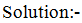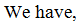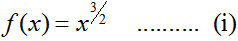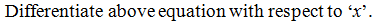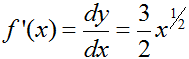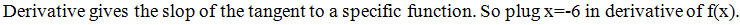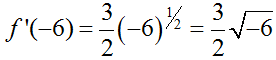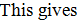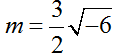Step 2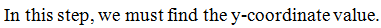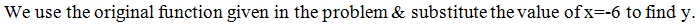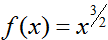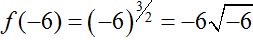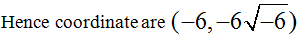...

### Want to see the full answer?

See Solution

#### Want to see this answer and more?

Solutions are written by subject experts who are available 24/7. Questions are typically answered within 1 hour.*

See Solution
*Response times may vary by subject and question.
Tagged in